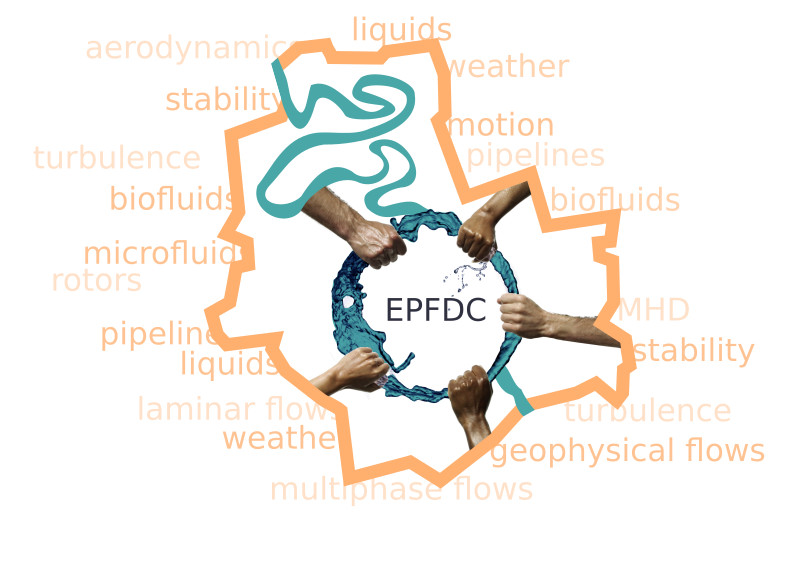# 8th European PostgraduateFluid Dynamics Conference

## 6th – 9th July 2016Warsaw, Poland

Back to programme

# Anisotropic advection of a passive vector field by the turbulent velocity flow with finite correlation time

N. V. Antonov1, N. M. Gulitskiy1
1Saint Petersburg State University
The generalization of the problem, considered in [Phys. Rev. E 91, 013002 (2015)], to the finite correlation time of the environment (velocity) field is studied. Inertial-range asymptotic behavior of a vector (e.g., magnetic) field, passively advected by a strongly anisotropic turbulent flow, is investigated by means of the field theoretic renormalization group and the operator product expansion. The inertial-range behavior of the model is described by two regimes (the limits of vanishing or infinite correlation time) that correspond to non-trivial fixed points of the RG equations. In contrast to the well-known isotropic Kraichnan’s model, where various correlation functions exhibit anomalous scaling behavior with infinite sets of anomalous exponents, here the dependence on the integral turbulence scale L has a logarithmic behavior. Due to the presence of anisotropy in the model all multiloop diagrams are equal to zero, thus, this result is
Keywords: Turbulence, Magnetohydrodynamics, Renormalization group